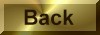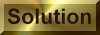Practice Problem 4

Calculate the standard-state entropy of reaction for the following reactions and explain the sign ofS° for each reaction.

(a) Hg(lHg(g)

(b) 2NO2(g)N2O4(g)

(c) N2(g) + O2(g)2NO(g)

(a)    98.94 J/K, the sign ofSo is positive.

(b)  -175.83 J/K, the sign ofSo is negative.

(c)     24.77 J/K, the sign ofSo for this reaction is small but positive.